# A Brief Introduction to Chopper Circuits

As the invention and use of technological devices are increasing, our need for electricity is also increasing. To meet this need of continuous electricity various methods and systems are being introduced. Among the gadgets and devices we use, some are powered by AC current while some are of DC powered. Not all devices require the same amount of power to operate. But the power given to the households through main power supply is AC and of a fixed amount of about 240v. Then to operate devices that work on DC current some converters are required. To use only a small required amount of power from the 240V supply another type of circuit namely Chopper circuit is required.

## What is a Chopper Circuit?

Chopper circuits are known as DC to DC converters. Similar to the transformers of the AC circuit, choppers are used to step up and step down the DC power. They change the fixed DC power to variable DC power. Using these, DC power supplied to the devices can be adjusted to the required amount.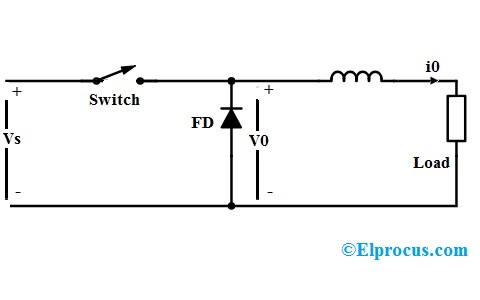Chopper Circuit

### Principle of Operation

The principle of operation of chopper can be understood from the circuit diagram below. The circuit consists of a semiconductor diode, resistor, and a load. For all type of chopper circuit, the output voltage value is controlled by periodic closing and opening of the switches used in the circuit.

The chopper can be viewed as an ON/OFF switch that can rapidly connect or disconnect the source to load connection. Continuous DC is given as source to the chopper as Vs and chopped DC is obtained across the load as V0.

### Output Voltage and Current Waveforms

Above are the output voltage and current waveforms of a chopper circuit. From the voltage waveform, it can be seen that during the period of TON the load voltage V0 is equal to the source voltage Vs. But when the interval TOFF occurs, the DC voltage step downs to zero, thus making the load short-circuited.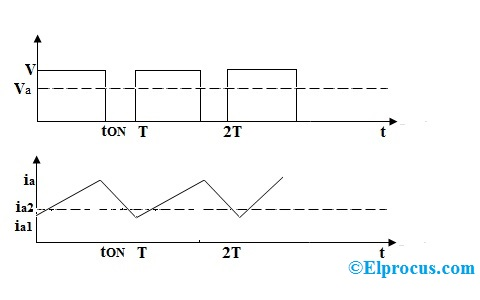Output Voltage and Current Waveforms

In the current waveform, it can be seen that during the interval TON the load current rises to the maximum value. During the interval TOFF, the load current decays. In TOFF condition the chopper is off so, the load voltage becomes zero. But load current flows through the diode FD, making the load short-circuited.

Thus, the chopped DC voltage is produced at the load. The current waveform is continuous which rises during TON state and decays during TOFF state.

## Classification of Chopper

Based on their operation principle and type of source voltage chopper are of various types. The main classification of the chopper is DC chopper and AC Link chopper. Based on the commutation process they are classified as a natural commutated chopper and forced commutated chopper.

Forced commutated chopper is further classified as Jones chopper, Morgan chopper. Based on output voltage values choppers are classified as a step down the chopper, step up chopper, step-up/down chopper. Based on the power loss occurred at switching time choppers are classified as Hard switched and soft switched.

### 1). AC Link Chopper

In this classification of the chopper, the voltage inversion takes place. Here the DC voltage is converted into AC with the help of an inverter. Now this AC is passed through a step-down or step-up transformers. The output from the transformers is again converted into DC by a rectifier. AC link choppers are very bulky and occupy a large amount of space.

### 2).DC Chopper

DC chopper works on DC voltage. They work as a step up and step down transformers on DC voltage. They can convert the steady constant DC voltage to a higher value or lower value based on their type.

DC choppers are more efficient, speed and optimized devices. These can be incorporated on electronic chips. They provide smooth control over the DC voltage.

### Different Types of Chopper Circuits

The main element based on which choppers are categorized is the semiconductor used in the chopper circuit. Based on the positioning of this semiconductor, choppers can be made to work in any of the four quadrant conditions. Depending on the quadrant of operation choppers are categorized as Type A, B, C, D, and E

• Type A chopper works in the first quadrant. In this chopper, the voltage and current both are positive and flows in the same direction. Power from source to load and the average output voltage is less than input DC voltage.
• Type B chopper works in the second quadrant. Here the load voltage is positive and current is negative. Power flows from load to source. This chopper is also known as a step-up chopper.
• Type C chopper is formed by parallel connection of Type A and Type B choppers.
• Type D chopper is the two quadrant type B chopper and Type E chopper is the fourth gradient chopper.

#### Step Up Chopper

Step-up chopper works as a step-up transformer on DC current. This chopper is used when the output DC voltage has to be made higher than the input voltage.

The working principle of a step up chopper can be explained from the above diagram. In the circuit, a large inductor L is connected in series to the supply voltage. Capacitor maintains the continuous output voltage to the load. The diode prevents the flow of current from load to source.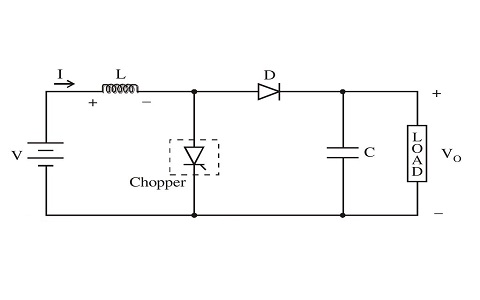Step up Chopper

When the chopper is ON, supply voltage VS is applied to the load .i.e. V0 = VS and inductor starts storing energy. At this condition load current raises from Imin to Imax.

When the chopper is switched OFF, the supply voltage takes the path from L – D – Load – VS. During this period the inductor discharges the stored e.m.f through diode D to the load. Thus the total voltage at the load V0 = VS + Ldi/dt which s greater than the input voltage. Current changes from Imax to Imin.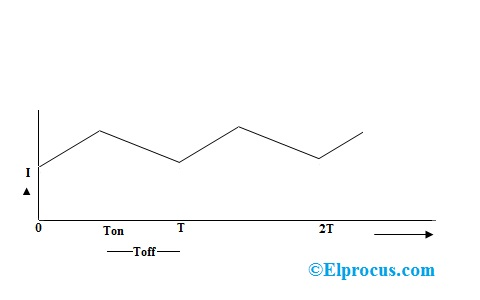Step up Chopper Current Waveform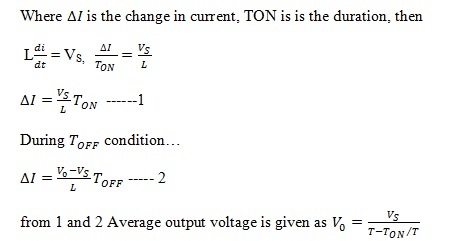Step up Chopper Equations

Step –up chopper is also known as Boost choppers. Applications of the step up choppers include battery charging and as a voltage booster.

### Applications of Chopper

DC to DC converters are applied for many applications such as in

• Switched Mode Power Supply System.
• in DC motors as speed controllers.
• DC voltage boosters.
• Battery chargers.
• Railway systems.
• Electric cars etc…

Choppers are used in signal processing systems also. In choppers, the output voltage can be controlled using many different techniques such as Pulse width modulation, Frequency modulation, Variable frequency, variable pulse width, CLC control, etc. Which of these methods are found efficient for chopper circuit in signal processing?